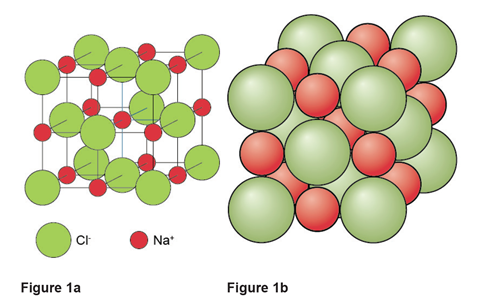Try this explainer to help students get to grips with unit cells and the structure of crystals as part of their preparation for the Chemistry Olympiad

While crystal unit cells have featured in past Olympiad papers, the topic is often less familiar to students at the time they take part in the competition. This explainer provides an introduction to unit cells in crystalline solids to consolidate students’ understanding of the key ideas.

Students read the introduction, before taking a look at a worked example of a past Olympiad question on the topic. They then have a go at answering a past question themselves, before checking their answers.

You can download the unit cells explainer as a PDF using the following link. The introduction to the topic is also reproduced below.

## Introducing crystal structures

Crystals are solids in which the particles have a regular arrangement held together by forces of attraction. The exact nature of these forces of attraction depends on the type of particles in the crystal. The highly ordered particles form a three dimensional structure known as a crystal lattice.

There are four basic crystal types: ionic, metallic, molecular and macromolecular.

In ionic crystals, such as sodium chloride, the particles are ions held in a lattice structure by strong electrostatic forces of attraction between the oppositely charged ions.

Metallic crystals exist as a lattice of positively charged metal ions surrounded by a sea of delocalised electrons. The lattice of metal ions is held in position by the strong electrostatic force of attraction between the positive metal ion and the delocalised electrons.

Molecular crystals consist of a lattice of simple covalent molecules held in a fixed position by intermolecular forces. Iodine is an example of a molecular crystal in which diatomic iodine, I2, molecules are held in position by the Van der Waals forces between the iodine molecules.

Finally, macromolecular crystals consist of atoms covalently bonded together to form one huge three dimensional crystal structure. Examples of macromolecular crystals include diamond and graphite.

## Unit cells

A unit cell is the smallest unit that when stacked together repeatedly without any gaps can reproduce the entire crystal.

The unit cell for sodium chloride is shown in figure 1. In figure 1a the green and red spheres represent the centre of the Cl and Na+ ions respectively. Figure 1b provides a more realistic representation with the sodium and chloride ions touching each other along the cell edges.Figure 1: two different representations of the unit cell for sodium chloride.

In both these representations of the unit cell it is important to note that the whole particle is not necessarily in the unit cell. A particle at a corner or on a face or an edge is shared by neighbouring unit cells so in a crystal only a fraction of it belongs to the unit cell in question:

• A particle at a corner is shared by eight neighbouring unit cells and hence just ⅛ of the particle is within the unit cell.
• A particle on an edge is shared by four neighbouring unit cells and so ¼ of it falls within the unit cell.
• A particle on a face is shared by two neighbouring unit cells and so only ½ of it falls within the unit cell.

The total number of particles in a unit cell can therefore be determined by counting the number of particles in each position of the unit cell (at a corner, edge, face or in the body) and multiplying by the fraction of each particle within the unit cell.

Table 1 shows such a calculation for the unit cell of sodium chloride. From the calculation we can see that each unit cell contains 4 Na+ ions and 4 Cl ions and hence the formula of sodium chloride is NaCl and it has no overall charge.

 Number of ions Na+ Cl– at 8 corners – 8 x  1/8 = 1 on 12 edges 12 x 1/4 = 3 – on 6 faces – 6 x 1/2 = 3 in the body of the cell 1 – Total 4 4

Knowing the numbers of each particle within a unit cell it is then possible to calculate other properties of the crystal.